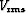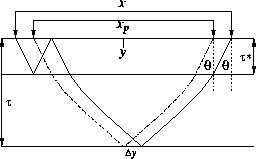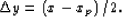Next: Differential Geometric Spreading Up: Particular Implementation of LSJIMP Previous: Amplitude corrections for pegleg

## Snell Resampling Normalizes AVO/Attenuation

Figureillustrates the fact that in a v(z) medium, there exists a single offset xp such that a pegleg with offset x and primary with offset xp are physically invariant with respect to AVO behavior and, assuming perfect elasticity in the top layer (often water), to anelastic attenuation. Since the pegleg multiple and primary in Figurehave the same emergence angle,, the time dip, or stepout'' of the two events is the same at x and xp. In Appendix, I derive the following expression for xp, where,, andwere defined in Section:

 schem-snell Figure 4 A primary and pegleg multiple with the same emergence angle () and midpoint (y). Note different offsets (x and xp) and a shift () in reflection point.(21)
Equation () defines, for a-order pegleg, a time-variable compression of the offset axis, which I denote Snell Resampling''. The name is derived from Ottolini's 1982 Snell Traces'' - a resampling of multi-offset reflection data along curves of constant stepout. Note that in a constant velocity medium,, and equation () reduces to the radial trace resampling used by Taner (1980) for the long-period deconvolution of peglegs.

Figureillustrates application of Snell Resampling to a synthetic CMP gather. From the Figure, we see that Snell Resampling is an important vehicle for the exploitation of the additional information contained in the multiples. Notice how energy from the multiples is spread into the coverage gaps at near offsets and at 1.0 km offset. Snell Resampling moves energy from the multiples to the offset corresponding to its reflection angle at the target.Figure 5
Snell Resampling demonstration. Left to right: a synthetic CMP gather after NMO for primaries and for first- and second-order peglegs, respectively. Snell Resampling (plus normalization to account for offset axis compression) was applied to the right two panels. The black lines illustrate the transformation's horizontal compression. The raw data has five unrecorded near offset traces and two dead traces at medium offsets.

Graphically (Figure), we may infer that the shift in midpoint,, of the reflection points of the primary and first-order pegleg is:(22)
As a function of time,decreases asymptotically to zero at infinite depth from a maximum of x/4 at the seabed. The deeper the reflector, the smallerbecomes. Similar expressions may be easily derived for multiples of other types and orders.

For peglegs arising from sub-seabed reflectors, the assumption of perfect elasticity for the multiple bounce breaks down. To some extent, however, the additional attenuation suffered by the multiple can, to first order, be treated as a decrease in reflection coefficient.Next: Differential Geometric Spreading Up: Particular Implementation of LSJIMP Previous: Amplitude corrections for pegleg
Stanford Exploration Project
5/30/2004